# 第3周

## 六、逻辑回归(Logistic Regression)

### 6.1 分类问题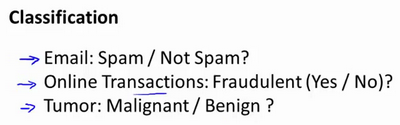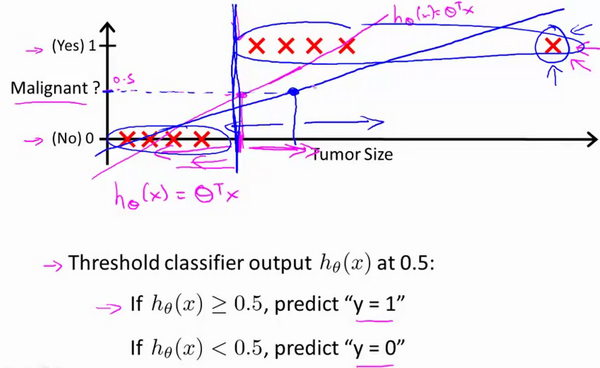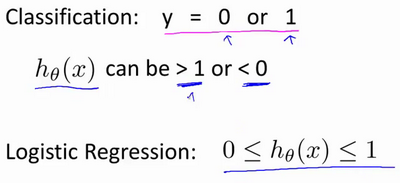### 6.2 假说表示${h_\theta}\left( x \right)>=0.5$时，预测 $y=1$

${h_\theta}\left( x \right)<0.5$时，预测 $y=0$python代码实现：

import numpy as np    def sigmoid(z):       return 1 / (1 + np.exp(-z))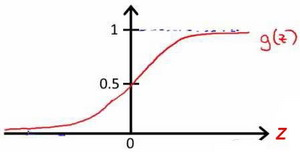$h_\theta \left( x \right)$的作用是，对于给定的输入变量，根据选择的参数计算输出变量=1的可能性（estimated probablity）即$h_\theta \left( x \right)=P\left( y=1|x;\theta \right)$ 例如，如果对于给定的$x$，通过已经确定的参数计算得出$h_\theta \left( x \right)=0.7$，则表示有70%的几率$y$为正向类，相应地$y$为负向类的几率为1-0.7=0.3。

### 6.3 判定边界${h_\theta}\left( x \right)>=0.5$时，预测 $y=1$

${h_\theta}\left( x \right)<0.5$时，预测 $y=0$

$z=0$$g(z)=0.5$

$z>0$$g(z)>0.5$

$z<0$$g(z)<0.5$

$z={\theta^{T}}x$ ，即： ${\theta^{T}}x>=0$ 时，预测 $y=1$ ${\theta^{T}}x<0$ 时，预测 $y=0$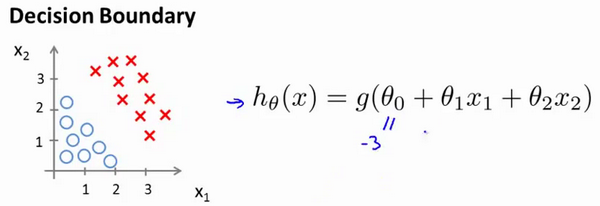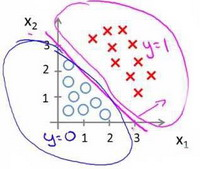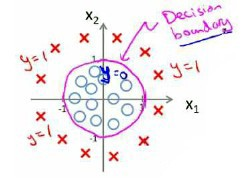### 6.4 代价函数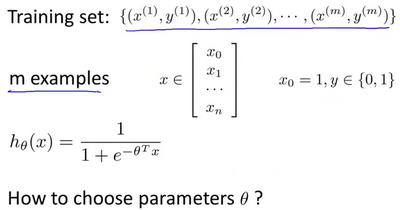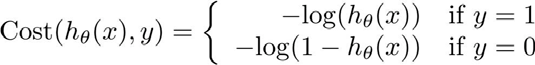${h_\theta}\left( x \right)$$Cost\left( {h_\theta}\left( x \right),y \right)$之间的关系如下图所示：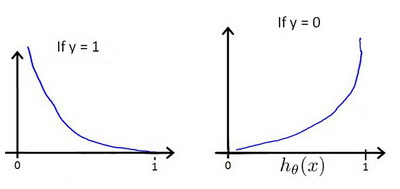Python代码实现：

xxxxxxxxxximport numpy as np    def cost(theta, X, y):      theta = np.matrix(theta)  X = np.matrix(X)  y = np.matrix(y)  first = np.multiply(-y, np.log(sigmoid(X* theta.T)))  second = np.multiply((1 - y), np.log(1 - sigmoid(X* theta.T)))  return np.sum(first - second) / (len(X))

Repeat { $\theta_j := \theta_j - \alpha \frac{\partial}{\partial\theta_j} J(\theta)$ (simultaneously update all ) }

Repeat { $\theta_j := \theta_j - \alpha \frac{1}{m}\sum\limits_{i=1}^{m}{{\left( {h_\theta}\left( \mathop{x}^{\left( i \right)} \right)-\mathop{y}^{\left( i \right)} \right)}}\mathop{x}_{j}^{(i)}$ (simultaneously update all ) }

$J\left( \theta \right)=-\frac{1}{m}\sum\limits_{i=1}^{m}{[{{y}^{(i)}}\log \left( {h_\theta}\left( {{x}^{(i)}} \right) \right)+\left( 1-{{y}^{(i)}} \right)\log \left( 1-{h_\theta}\left( {{x}^{(i)}} \right) \right)]}$ 考虑： ${h_\theta}\left( {{x}^{(i)}} \right)=\frac{1}{1+{{e}^{-{\theta^T}{{x}^{(i)}}}}}$ 则： ${{y}^{(i)}}\log \left( {h_\theta}\left( {{x}^{(i)}} \right) \right)+\left( 1-{{y}^{(i)}} \right)\log \left( 1-{h_\theta}\left( {{x}^{(i)}} \right) \right)$ $={{y}^{(i)}}\log \left( \frac{1}{1+{{e}^{-{\theta^T}{{x}^{(i)}}}}} \right)+\left( 1-{{y}^{(i)}} \right)\log \left( 1-\frac{1}{1+{{e}^{-{\theta^T}{{x}^{(i)}}}}} \right)$ $=-{{y}^{(i)}}\log \left( 1+{{e}^{-{\theta^T}{{x}^{(i)}}}} \right)-\left( 1-{{y}^{(i)}} \right)\log \left( 1+{{e}^{{\theta^T}{{x}^{(i)}}}} \right)$

​xfunction [jVal, gradient] = costFunction(theta)​    jVal = [...code to compute J(theta)...];    gradient = [...code to compute derivative of J(theta)...];    end    options = optimset('GradObj', 'on', 'MaxIter', '100');    initialTheta = zeros(2,1);    [optTheta, functionVal, exitFlag] = fminunc(@costFunction, initialTheta, options);

### 6.5 简化的成本函数和梯度下降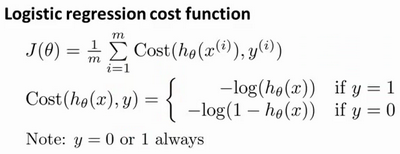$Cost\left( {h_\theta}\left( x \right),y \right)=-y\times log\left( {h_\theta}\left( x \right) \right)-(1-y)\times log\left( 1-{h_\theta}\left( x \right) \right)$ 即，逻辑回归的代价函数： $Cost\left( {h_\theta}\left( x \right),y \right)=-y\times log\left( {h_\theta}\left( x \right) \right)-(1-y)\times log\left( 1-{h_\theta}\left( x \right) \right)$ $=-\frac{1}{m}\sum\limits_{i=1}^{m}{[{{y}^{(i)}}\log \left( {h_\theta}\left( {{x}^{(i)}} \right) \right)+\left( 1-{{y}^{(i)}} \right)\log \left( 1-{h_\theta}\left( {{x}^{(i)}} \right) \right)]}$ 根据这个代价函数，为了拟合出参数，该怎么做呢？我们要试图找尽量让$J\left( \theta \right)$ 取得最小值的参数$\theta$ $\underset{\theta}{\min }J\left( \theta \right)$ 所以我们想要尽量减小这一项，这将我们将得到某个参数$\theta$ 如果我们给出一个新的样本，假如某个特征 $x$，我们可以用拟合训练样本的参数$\theta$，来输出对假设的预测。 另外，我们假设的输出，实际上就是这个概率值：$p(y=1|x;\theta)$，就是关于 $x$$\theta$为参数，$y=1$ 的概率，你可以认为我们的假设就是估计 $y=1$ 的概率，所以，接下来就是弄清楚如何最大限度地最小化代价函数$J\left( \theta \right)$，作为一个关于$\theta$的函数，这样我们才能为训练集拟合出参数$\theta$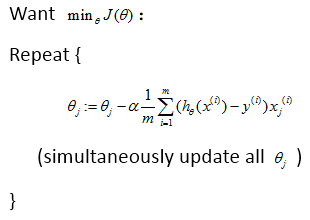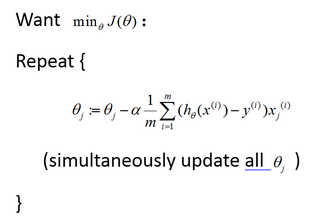${\theta_j}:={\theta_j}-\alpha \frac{1}{m}\sum\limits_{i=1}^{m}{({h_\theta}({{x}^{(i)}})-{{y}^{(i)}}){{x}_{j}}^{(i)}}$来同时更新所有$\theta$的值。

${h_\theta}\left( x \right)={\theta^T}X={\theta_{0}}{x_{0}}+{\theta_{1}}{x_{1}}+{\theta_{2}}{x_{2}}+...+{\theta_{n}}{x_{n}}$

${h_\theta}\left( x \right)=\frac{1}{1+{{e}^{-{\theta^T}X}}}$

### 6.6 高级优化Octave 有一个非常理想的库用于实现这些先进的优化算法，所以，如果你直接调用它自带的库，你就能得到不错的结果。我必须指出这些算法实现得好或不好是有区别的，因此，如果你正在你的机器学习程序中使用一种不同的语言，比如如果你正在使用CC++Java等等，你可能会想尝试一些不同的库，以确保你找到一个能很好实现这些算法的库。因为在L-BFGS或者等高线梯度的实现上，表现得好与不太好是有差别的，因此现在让我们来说明：如何使用这些算法：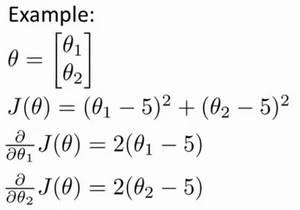$\frac{\partial }{\partial {{\theta }_{1}}}J(\theta)=2({{\theta }_{1}}-5)$

$\frac{\partial }{\partial {{\theta }_{2}}}J(\theta)=2({{\theta }_{2}}-5)$

xxxxxxxxxxfunction [jVal, gradient]=costFunction(theta)    　　jVal=(theta(1)-5)^2+(theta(2)-5)^2;    　　gradient=zeros(2,1);    　　gradient(1)=2*(theta(1)-5);    　　gradient(2)=2*(theta(2)-5);    end

xxxxxxxxxxoptions=optimset('GradObj','on','MaxIter',100);​initialTheta=zeros(2,1);    [optTheta, functionVal, exitFlag]=fminunc(@costFunction, initialTheta, options);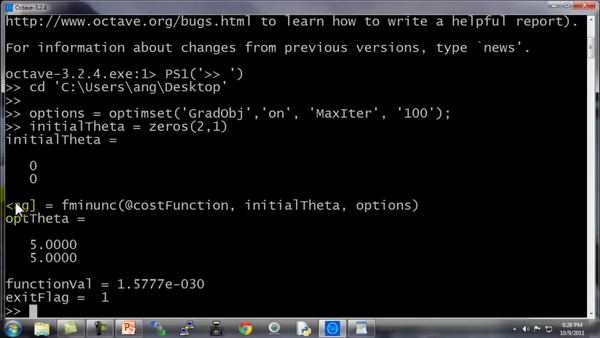### 6.7 多类别分类：一对多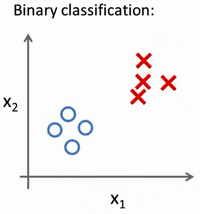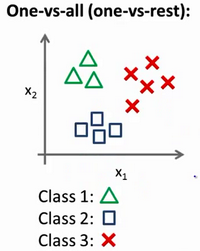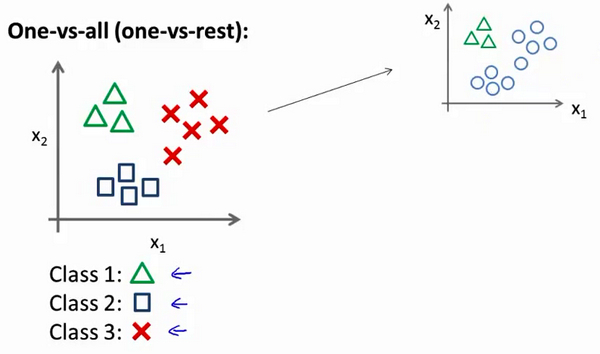## 七、正则化(Regularization)

### 7.1 过拟合的问题1. 丢弃一些不能帮助我们正确预测的特征。可以是手工选择保留哪些特征，或者使用一些模型选择的算法来帮忙（例如PCA
2. 正则化。 保留所有的特征，但是减少参数的大小（magnitude）。

### 7.2 代价函数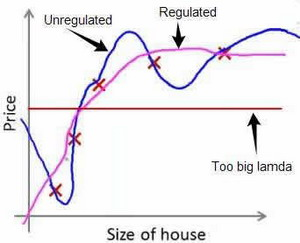### 7.3 正则化线性回归

$J\left( \theta \right)=\frac{1}{2m}\sum\limits_{i=1}^{m}{[({{({h_\theta}({{x}^{(i)}})-{{y}^{(i)}})}^{2}}+\lambda \sum\limits_{j=1}^{n}{\theta _{j}^{2}})]}$

$Repeat$ $until$ $convergence${

${\theta_0}:={\theta_0}-a\frac{1}{m}\sum\limits_{i=1}^{m}{(({h_\theta}({{x}^{(i)}})-{{y}^{(i)}})x_{0}^{(i)}})$

${\theta_j}:={\theta_j}-a[\frac{1}{m}\sum\limits_{i=1}^{m}{(({h_\theta}({{x}^{(i)}})-{{y}^{(i)}})x_{j}^{\left( i \right)}}+\frac{\lambda }{m}{\theta_j}]$

$for$ $j=1,2,...n$

}

${\theta_j}:={\theta_j}(1-a\frac{\lambda }{m})-a\frac{1}{m}\sum\limits_{i=1}^{m}{({h_\theta}({{x}^{(i)}})-{{y}^{(i)}})x_{j}^{\left( i \right)}}$ 可以看出，正则化线性回归的梯度下降算法的变化在于，每次都在原有算法更新规则的基础上令$\theta$值减少了一个额外的值。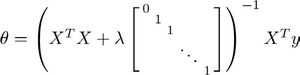### 7.4 正则化的逻辑回归模型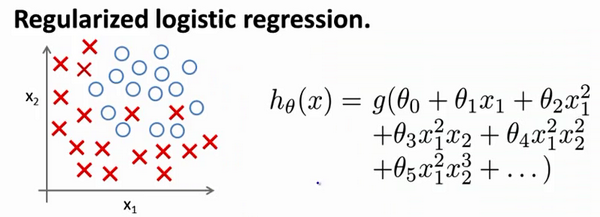$J\left( \theta \right)=\frac{1}{m}\sum\limits_{i=1}^{m}{[-{{y}^{(i)}}\log \left( {h_\theta}\left( {{x}^{(i)}} \right) \right)-\left( 1-{{y}^{(i)}} \right)\log \left( 1-{h_\theta}\left( {{x}^{(i)}} \right) \right)]}+\frac{\lambda }{2m}\sum\limits_{j=1}^{n}{\theta _{j}^{2}}$

Python代码：

xxxxxxxxxximport numpy as np​def costReg(theta, X, y, learningRate):    theta = np.matrix(theta)    X = np.matrix(X)    y = np.matrix(y)    first = np.multiply(-y, np.log(sigmoid(X*theta.T)))    second = np.multiply((1 - y), np.log(1 - sigmoid(X*theta.T)))    reg = (learningRate / (2 * len(X))* np.sum(np.power(theta[:,1:theta.shape],2))    return np.sum(first - second) / (len(X)) + reg

$Repeat$ $until$ $convergence${

${\theta_0}:={\theta_0}-a\frac{1}{m}\sum\limits_{i=1}^{m}{(({h_\theta}({{x}^{(i)}})-{{y}^{(i)}})x_{0}^{(i)}})$

${\theta_j}:={\theta_j}-a[\frac{1}{m}\sum\limits_{i=1}^{m}{({h_\theta}({{x}^{(i)}})-{{y}^{(i)}})x_{j}^{\left( i \right)}}+\frac{\lambda }{m}{\theta_j}]$

$for$ $j=1,2,...n$

}

1. 虽然正则化的逻辑回归中的梯度下降和正则化的线性回归中的表达式看起来一样，但由于两者的${h_\theta}\left( x \right)$不同所以还是有很大差别。
2. ${\theta_{0}}$不参与其中的任何一个正则化。